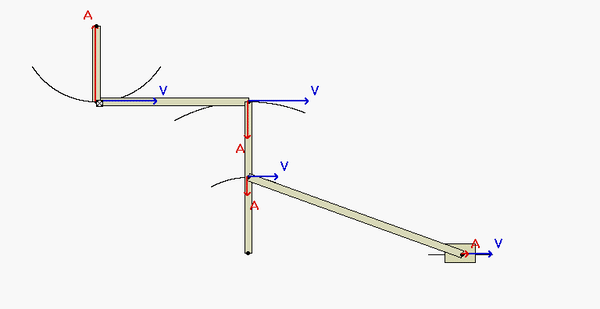# Homework H2.J - Sp23

 Problem statement Solution videoBefore starting this problem, make note of the type of motion for each component in the mechanism:

• Links OA and BC are in pure rotation about their centers of rotation O and C, respectively. From this, we know that the paths of points A, B and C are circular, as seen in the animation below.
• Block E is in pure translation.
• Links AB and DE have both translational and rotation components of motion.Question: What are the locations of the instant centers (ICs) of AB and DE at this instant? Reflect back on the observations above in answering this. What do these locations say about the angular velocities of AB and DE at this position?HINTS:
Once you have found the angular velocities for all of the links, you can then tackle the acceleration analysis.

• For finding the angular acceleration of links AB and BC, use the following rigid body acceleration equations:
aA = aO + αOA x rA/O - ωOA2rA/O
aB = aC + αBC x rB/C - ωBC2rB/C
aB = aA + αAB x rB/A - ωAB2rB/A
This will give you the equations that you need to solve for the desired angular accelerations.
• Repeat the above for link DE to determine its angular acceleration:
aD = aC + αBC x rD/C - ωBC2rD/C
aE = aD + αDE x rE/D - ωDE2rE/D

## 25 thoughts on “Homework H2.J - Sp23”

1.eaub says:

for the equation of a_e, is there a typo when it says r_e/o? shouldn't/couldn't it be r_e/d?

1.CMK says:

You are correct. I have fixed the typo. Thanks for letting us know.

2.lin1449 says:

are wab and wde equal to zero? since the ICs are parallel?

1.CMK says:

Yes, that is correct on both counts.

1.Arul Verma says:

If this is the case and vE=wDE * rDE, how can I find vE? Because wDE * rDE would give 0...?

1.CMK says:

omega_DE = 0 tells us that all points on DE have the same velocity. Therefore, vE = vD = L*omega_BC.

The equation that you quote above, vE=wDE * rDE, is not valid because vD is not zero.

3.Gage Alan Wilson Ernsberger says:

Is angular acceleration 0 when angular velocity is 0?

1.CMK says:

Nope. omega is typically zero only for the one instant.

4.Adam Joseph Irons says:

Are the angular velocities of link BC and link DC equal to each other?

1.CMK says:

There is only one link, BD, not two.

5.Vishwesh Krishnamurthy says:

Should we write our answers in terms of given variables? I just wanted to double check

1.CMK says:

In terms of, at most, omega_OA and L.

6.Jacob Russell Bunton says:

In the first acceleration equation a_A = a_O + α_OA x r_A/O - ω_OA^2(r_A/O). Does a_O = 0, since its velocity (v_O) is constantly 0 because its pinned? And does α_OA equal 0, since w_OA is constant as stated in the problem?

1.CMK says:

Yes, for both questions.

7.Alexander Edward Kennon says:

What should the answers to the problem be in terms of?

1.CMK says:

In terms of, at most, omega_OA and L.

8.Michael Bradley Mayhew says:

When looking at omega_OA compared to omega_BC, do we need to care about the directional sign since we are going to use it to just find the speed of E? I end up getting -Lomega_OA for V_E if I include the sign for direction of rotation while substituting, but in the animation V_E is going the same direction as link OA.

1.Michael Bradley Mayhew says:

I guess my question boils down to should I keep omega_BC equal to -omega_OA or do I disregard the different rotation directions and have the prior equal +omega_OA?

1.Eva Katherine Lynch says:

What I did was use Va = Vb ---> Vc (in terms of Vb) then use the IC eqn (wR=V) for Vb which you can use to fin Wbc in terms of Woa. Also, my Wbc is positive.

2.CMK says:

MIchael: The instant center approach is based on speeds (unsigned numbers). Deal with positive values. Get the direction of the rotations by looking at your figure.

9.Charlotte says:

When looking at the link that includes B, D, and C, which link should we use when using the IC approach for v_D (in relation to the angular velocity of BC). Because D is in between B and C, would that equation be v_D = w_BC * BC or v_D = w_BC * DC. I know this has come up before I was just never sure of which one was correct

1.CMK says:

Once you have found w_BC, then use v_D = w_BC * DC.

The other option that you provide above, v_D = w_BC * BC, is not correct - that expression is for v_B, not v_D.

10.Phillip Eugene Holmes says:

Would the acceleration of point a only be on the j direction?

1.AJ Collins says:

Yes, the snapshot of the simulation shows that a_A is in the positive j_hat direction.

11.AJ Collins says:

When looking at the acceleration equations, I have found a value for the acceleration of A in terms of A and wOA, but now I am stuck on where to go from here. Looking at the two equations solving for a_B, there are three unknowns and two equations. Is there some inequality that I'm forgetting about or some way that I can write one variable in terms of another? Or should I set the two equations equal to one another and solve from there?